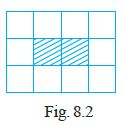#### Fill in the blanks to make the statement true.Use Fig. 8.2 (In which each square is of unit length) for questions 39 and 40:The ratio of the perimeter of the boundary of the shaded portion to the perimeter of the whole figure is _______.

The perimeter of the shaded portion = 6 units

The perimeter of the whole figure  = 14 units

The ratio of the perimeter of the boundary of the shaded portion to the perimeter of the whole figure is